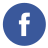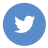### encrypt by java and decrypt by node

javanodejs

To encrypt a message using Java and decrypt it using Node.js, you can follow these steps:

1. Choose an encryption algorithm and a key. You will need to use the same algorithm and key for both the Java and Node.js programs. Some popular algorithms for symmetric encryption include AES and Blowfish.

2. In your Java program, use the Cipher class from the javax.crypto package to encrypt the message. Here’s an example of how you can do this:

``````import javax.crypto.Cipher;
import javax.crypto.spec.SecretKeySpec;

public class Main {
public static void main(String[] args) throws Exception {
// Set up the key and cipher
SecretKeySpec key = new SecretKeySpec("your-key-here".getBytes(), "AES");
Cipher cipher = Cipher.getInstance("AES");
cipher.init(Cipher.ENCRYPT_MODE, key);

// Encrypt the message
String message = "Hello, world!";
byte[] encryptedMessage = cipher.doFinal(message.getBytes());

// Convert the encrypted message to a hexadecimal string
String hexEncryptedMessage = bytesToHex(encryptedMessage);

System.out.println(hexEncryptedMessage);
}

private static String bytesToHex(byte[] bytes) {
StringBuilder result = new StringBuilder();
for (byte b : bytes) {
result.append(String.format("%02x", b));
}
return result.toString();
}
}``````

This code will encrypt the message “Hello, world!” using the AES algorithm and the key “your-key-here”, and output the encrypted message as a hexadecimal string.

1. In your Node.js program, use the crypto module to decrypt the message. Here’s an example of how you can do this:
``````const crypto = require('crypto');

function decrypt(key, encryptedMessage) {
// Convert the hexadecimal encrypted message back to a buffer
const buffer = Buffer.from(encryptedMessage, 'hex');

// Set up the decipher
const decipher = crypto.createDecipher('aes-256-cbc', key);

// Decrypt the message
let decryptedMessage = decipher.update(buffer);
decryptedMessage = Buffer.concat([decryptedMessage, decipher.final()]);

return decryptedMessage.toString();
}

const key = 'your-key-here';
const encryptedMessage = 'your-encrypted-message-here';
console.log(decrypt(key, encryptedMessage));``````

This code will decrypt the message using the AES algorithm and the key “your-key-here”, and output the decrypted message as a string.

Note that the key and the encrypted message must be transmitted securely between the Java and Node.js programs, or else an attacker could intercept them and decrypt the message.